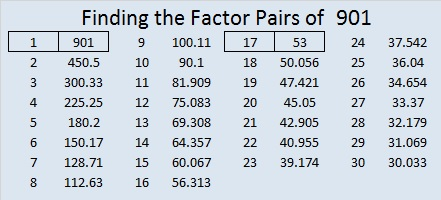# 901 and Level 4Print the puzzles or type the solution in this excel file: 10-factors-897-904

901 is the 25th centered triangular number because (23×24 + 24×25 + 25×26)/2 = 901. That is the same as saying that 901 is the sum of the 23rd, the 24th, and the 25th triangular numbers.

901 is the sum of two squares two different ways:

• 30² + 1² = 901
• 26² + 15² = 901

901 is the hypotenuse of FOUR Pythagorean triples:

• 60-899-901, calculated from 2(30)(1), 30² – 1², 30² + 1²
• 424-795-901, which is (8-15-17) times 53
• 451-780-901, calculated from 26² – 15², 2(26)(15), 26² + 15²
• 476-765-901, which is 17 times (28-45-53)

Two of those were primitives. That can only happen because ALL of 901’s prime factors are Pythagorean triple hypotenuses.

• 901 is a composite number.
• Prime factorization: 901 = 17 × 53
• The exponents in the prime factorization are 1 and 1. Adding one to each and multiplying we get (1 + 1)(1 + 1) = 2 × 2 = 4. Therefore 901 has exactly 4 factors.
• Factors of 901: 1, 17, 53, 901
• Factor pairs: 901 = 1 × 901 or 17 × 53
• 901 has no square factors that allow its square root to be simplified. √901 ≈ 30.016662This site uses Akismet to reduce spam. Learn how your comment data is processed.